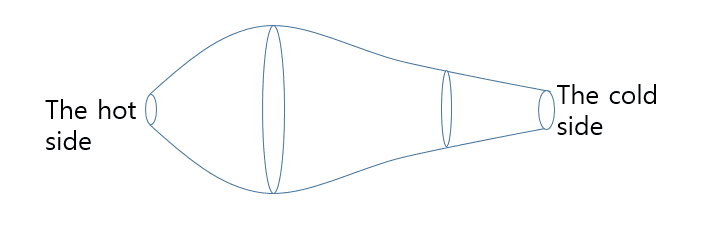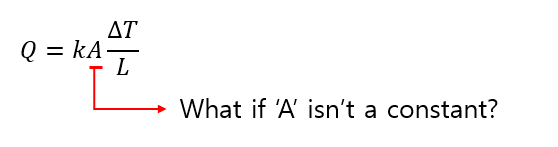# Rate of conduction heat transfer with different hot-side and cold-side areas

• emtae55

#### emtae55

When we measure 'the rate of conduction heat transfer'=Q , we assume that the hot side and the cold side's area are same. But if the both side's area is different to each other, how can i know the rate of conduction heat transfer?
like below figure.Would you like to help me?? Thanks.

Last edited:

Summary:: When we measure a thermal conductivity, we assume that the hot side and the cold side's area are same. But if the both side's area is different to each other, how can i know the thermal conductivity?

When we measure a thermal conductivity, we assume that the hot side and the cold side's area are same. But if the both side's area is different to each other, how can i know the thermal conductivity?
Would you like to help me?? Thanks.
Please provide a diagram illustrating the kind of geometric arrangement you are referring to.

Summary:: When we measure a thermal conductivity, we assume that the hot side and the cold side's area are same. But if the both side's area is different to each other, how can i know the thermal conductivity?
...
Thermal conductivity remains the same for same material.
In your case, what changes is the heat flux, which dependds on cross section or area.

https://en.m.wikipedia.org/wiki/Heat_flux

•russ_watters
Please provide a diagram illustrating the kind of geometric arrangement you are referring to.
I edited it.

As said, conductivity is a property of the material, not iys geometry.
So you prob. meant conductance or resistance.
Just calculate the reisitance of a differential cross-sectional volume & integrate.

•russ_watters
As said, conductivity is a property of the material, not iys geometry.
So you prob. meant conductance or resistance.
Just calculate the reisitance of a differential cross-sectional volume & integrate.
@Chestermiller is the subject matter expert here, but it seems to me that you would need to make some assumptions of uniformity and directionality for the heat flow before a scalar figure for resistance across a cross-section becomes meaningful.

For a reasonably long and thin object, such assumptions may be reasonable, but it is good to be aware that one is making them.

•etotheipi, Chestermiller and russ_watters
@Chestermiller is the subject matter expert here, but it seems to me that you would need to make some assumptions of uniformity and directionality for the heat flow before a scalar figure for resistance across a cross-section becomes meaningful.

For a reasonably long and thin object, such assumptions may be reasonable, but it is good to be aware that one is making them.
For the figure the OP provided, what i suggested would be appropriate.

Of course, you can be infInitely picky, such as heat lost/gained along the figure - but this is supposed to be introductory physIcs. Sturm-Liouville is not yet encountered, for example.

Oh, I made a mistake. What i meant was 'not' a conductivity but 'the rate of conduction heat transfer'
Sorry :(
I'll edit my subject.

I agree with @jbriggs444. If the cross sectional area were changing very gradually (like toward the right side of the figure, but not like toward the left side of the figure in post #1), you could write $$Q=kA(x)\frac{dT}{dx}$$where Q is the rate of heat flow (independent of x) and A(x) is the local cross sectional area. This would integrate to $$Q=k\frac{\Delta T}{\int_0^L{\frac{dx}{A(x)}}}$$

If the geometry does not vary gradually, one would have to solve the 2D or 3D heat conduction equation, a partial differential equation.

•etotheipi